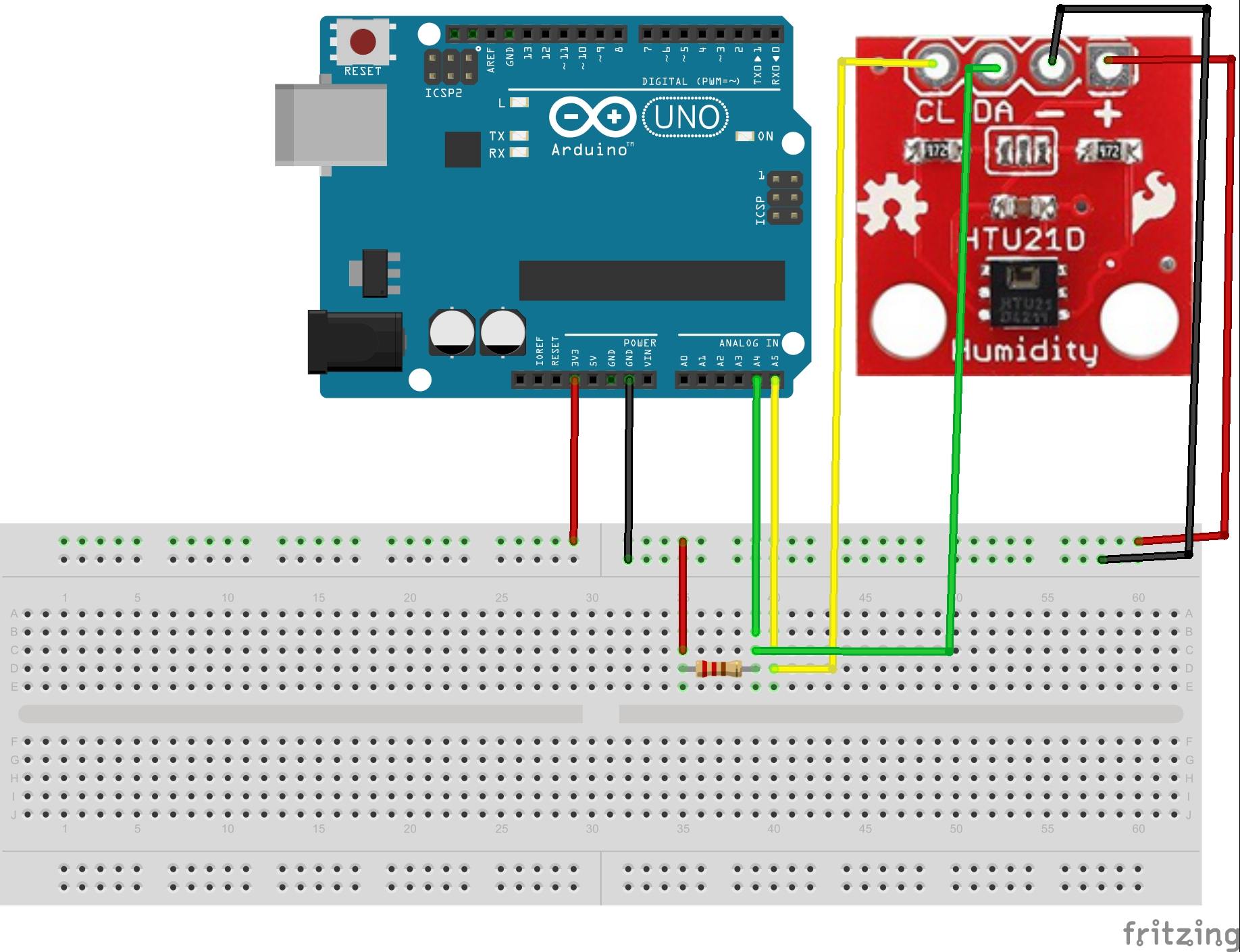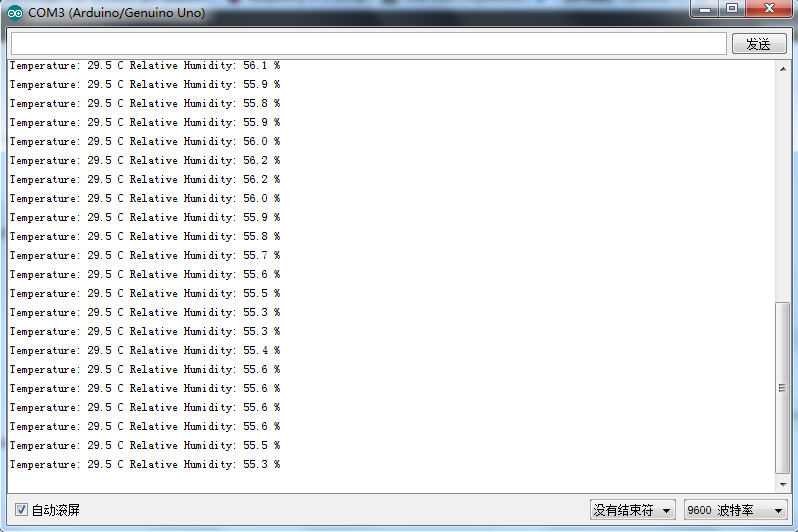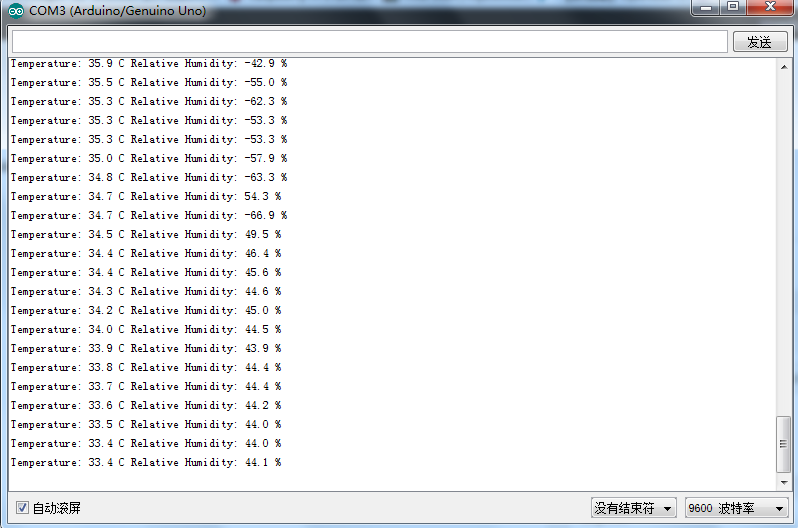HTU21D湿度/温度 センサーはIIC ポートを搭載、入力電圧は3.3Vとなります。このプロジェクトでは、Arduinoボードを使用して、
センサーからの湿度/温度データを獲得し、 ArduinoIDEのserial モニター窓に表示します。

Arduino UNO R3ボード x 1

USB ケーブルx 1

HTU21D センサーx 1

10k ohm 抵抗x 1

Circuit Graph:サンプルコード:

#include

#define TRIGGER_TEMP_MEASURE_HOLD  0xE3
#define TRIGGER_HUMD_MEASURE_HOLD  0xE5
#define TRIGGER_TEMP_MEASURE_NOHOLD  0xF3
#define TRIGGER_HUMD_MEASURE_NOHOLD  0xF5
#define WRITE_USER_REG  0xE6
#define SOFT_RESET  0xFE

byte sensorStatus;

void setup()
{
Serial.begin(9600);
Serial.println(“HTU21D Example!”);

Wire.begin();
}

void loop()
{

float temperature = calc_temp(rawTemperature);
float relativeHumidity = calc_humidity(rawHumidity); //Turn the humidity signal into actual humidity

Serial.print(“Temperature: “);
Serial.print(temperature, 1);
Serial.print(” C”);
Serial.print(” Relative Humidity: “);
Serial.print(relativeHumidity, 1);
Serial.print(” %”);
Serial.println();
delay(1000);
}

{
//Request the temperature
Wire.write(TRIGGER_TEMP_MEASURE_NOHOLD);
Wire.endTransmission();

//Wait for sensor to complete measurement
delay(60); //44-50 ms max – we could also poll the sensor

//Comes back in three bytes, data(MSB) / data(LSB) / CRC

//Wait for data to become available
int counter = 0;
while(Wire.available() < 3)
{
counter++;
delay(1);
if(counter > 100) return 998; //Error out
}

unsigned char msb, lsb, crc;
crc = Wire.read(); //We don’t do anything with CRC for now

unsigned int temperature = ((unsigned int)msb << 8) | lsb;
temperature &= 0xFFFC; //Zero out the status bits but keep them in place

return temperature;
}

{
byte msb, lsb, checksum;

Wire.write(TRIGGER_HUMD_MEASURE_NOHOLD); //Measure humidity with no bus holding
Wire.endTransmission();

//Hang out while measurement is taken. 50mS max, page 4 of datasheet.
delay(55);

//Wait for data to become available
int counter = 0;
while(Wire.available() < 3)
{
counter++;
delay(1);
if(counter > 100) return 0; //Error out
}

unsigned int rawHumidity = ((unsigned int) msb << 8) | (unsigned int) lsb;
rawHumidity &= 0xFFFC; //Zero out the status bits but keep them in place

return(rawHumidity);
}

//Given the raw temperature data, calculate the actual temperature
float calc_temp(int SigTemp)
{
float tempSigTemp = SigTemp / (float)65536; //2^16 = 65536
float realTemperature = -46.85 + (175.72 * tempSigTemp); //From page 14

return(realTemperature);
}

//Given the raw humidity data, calculate the actual relative humidity
float calc_humidity(int SigRH)
{
float tempSigRH = SigRH / (float)65536; //2^16 = 65536
float rh = -6 + (125 * tempSigRH); //From page 14

return(rh);
}

{
byte userRegister;

//Request the user register
Wire.endTransmission();

return(userRegister);
}

//Write to the user register
//NOTE: We disable all bits except for measurement resolution
//Bit 7 & 0 = Measurement resolution
//Bit 6 = Status of battery
//Bit 5/4/3 = Reserved
//Bit 2 = Enable on-board heater
//Bit 1 = Disable OTP reload
void write_user_register(byte thing_to_write)
{
byte userRegister = read_user_register(); //Go get the current register state
userRegister &= 0b01111110; //Turn off the resolution bits
thing_to_write &= 0b10000001; //Turn off all other bits but resolution bits
userRegister |= thing_to_write; //Mask in the requested resolution bits

//Request a write to user register
Wire.write(WRITE_USER_REG); //Write to the user register
Wire.write(userRegister); //Write to the data
Wire.endTransmission();
}

//Give this function the 2 byte message (measurement) and the check_value byte from the HTU21D
//If it returns 0, then the transmission was good
//If it returns something other than 0, then the communication was corrupted
//From: http://www.nongnu.org/avr-libc/user-manual/group__util__crc.html
//POLYNOMIAL = 0x0131 = x^8 + x^5 + x^4 + 1 : http://en.wikipedia.org/wiki/Computation_of_cyclic_redundancy_checks
#define SHIFTED_DIVISOR 0x988000 //This is the 0x0131 polynomial shifted to farthest left of three bytes

unsigned int check_crc(uint16_t message_from_sensor, uint8_t check_value_from_sensor)
{
//Test cases from datasheet:
//message = 0xDC, result is 0x79
//message = 0x683A, result is 0x7C
//message = 0x4E85, result is 0x6B

uint32_t remainder = (uint32_t)message_from_sensor << 8; //Pad with 8 bits because we have to add in the result/check value
remainder |= check_value_from_sensor; //Add on the check value

uint32_t divsor = (uint32_t)SHIFTED_DIVISOR;

for (int i = 0 ; i < 16 ; i++) //Operate on only 16 positions of max 24. The remaining 8 are our remainder and should be zero when we're done.
{
//Serial.print(“remainder:  “);
//Serial.println(remainder, BIN);
//Serial.print(“divsor:     “);
//Serial.println(divsor, BIN);
//Serial.println();

if( remainder & (uint32_t)1<<(23 - i) ) //Check if there is a one in the left position
remainder ^= divsor;

divsor >>= 1; //Rotate the divsor max 16 times so that we have 8 bits left of a remainder
}

return remainder;
}

Arduino IDEのserial モニターを開けて、 周囲環境の温度と湿度データが表示します：センサーを火焰に近づいてくると温度数値は高くなります: